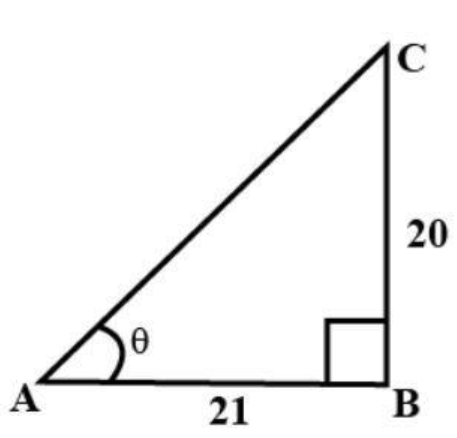QuestionAnswers

# If $\tan \theta = \dfrac{{20}}{{21}}$, show that$\dfrac{{1 - \sin \theta + \cos \theta }}{{1 + \sin \theta + \cos \theta }} = \dfrac{3}{7}$.Verified
155.4k+ views
Hint: Here we first draw the diagram of a triangle in which we show the value of trigonometric ratio as we know tan is the ratio of perpendicular to base of a right angle triangle. By the help of that triangle we find out the other ratio to prove our question.

Here in the question it is given that$\tan \theta = \dfrac{{20}}{{21}}$. And as we know tan is the ratio of perpendicular to base of a right angle triangle.
Let us consider the right triangle ABC (see fig), with base AB=21 and the perpendicular BC=20.By Pythagoras theorem, we have
$A{C^2} = A{B^2} + B{C^2}$ We use Pythagoras theorem in the right angle triangle to find out the third unknown term.
$\Rightarrow A{C^2} = A{B^2} + B{C^2} \\ \Rightarrow A{C^2} = {21^2} + {20^2} \\ \Rightarrow A{C^2} = 441 + 400 \\ \Rightarrow AC = \sqrt {841} \\$
$\therefore AC = 29$
And here for proving the question we have to find out the trigonometric ratios of sin and cos.
As we know sin is the ratio of perpendicular to hypotenuse of the right angle triangle. i.e. $\dfrac{{BC}}{{AC}} = \dfrac{{20}}{{29}}$
And we know cos is the ratio of base to hypotenuse of the right angle triangle. i.e. $\dfrac{{AB}}{{AC}} = \dfrac{{21}}{{29}}$
Here we use this condition for the angle$\theta$.
Now the value of $\sin \theta = \dfrac{{20}}{{29}}$ and $\cos \theta = \dfrac{{21}}{{29}}$ put these values in the question left hand side i.e. L.H.S.$\dfrac{{1 - \sin \theta + \cos \theta }}{{1 + \sin \theta + \cos \theta }}$
$\Rightarrow \dfrac{{1 - \dfrac{{20}}{{29}} + \dfrac{{21}}{{29}}}}{{1 + \dfrac{{20}}{{29}} + \dfrac{{21}}{{29}}}} = \dfrac{{\dfrac{{29 - 20 + 21}}{{29}}}}{{\dfrac{{29 + 20 + 21}}{{29}}}} = \dfrac{{\dfrac{{30}}{{29}}}}{{\dfrac{{70}}{{29}}}} \\ = \dfrac{{30}}{{29}} \times \dfrac{{29}}{{70}} = \dfrac{3}{7} \\$
Now here we see that the value of L.H.S. is equal to the right hand side R.H.S. i.e. $\dfrac{3}{7}$
Hence proved.

Note: Whenever we face such type of question the key concept for solving the question is whenever a trigonometric ratio is given for specific angle in the question then find out the other trigonometric ratio that are used for proving the question for that specific angle using Pythagoras theorem and using the ratio of other trigonometric function.